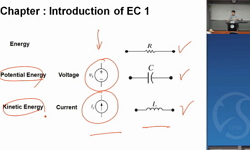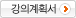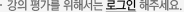### 주메뉴

### 회로이론2

• 성균관대학교
• 이명현• 주제분류
공학 >전기ㆍ전자 >전기/전자공학
• 강의학기
2016년 2학기
• 조회수
9,765
•
강의계획서1학기 회로이론 1에서 습득한 기본적인 회로 해석능력을 토대로, 비정현파 교류, 3상 회로 및 기본 회로의 과도 현상 등을 취급하는 능력을 습득한다. 아울러, 2단자망, 4단자망, 분포정수회로 등을 공부함으로써, 상급학년에서 이수하게 될 교과목들의 기초를 함양하고, 보편적인 회로 해석 능력을 배양시킨다.

#### 차시별 강의1.Review on Electrical Circuit I Review on Electrical Circuit I을 배운다2.The Sinusoidal Response The Sinusoidal Response를 배운다3.Calculation of complex number Calculation of complex number를 배운다4.Impedence and Reactance Impedence and Reactance를 배운다5.Combining Impedances in Parallel Combining Impedances in Parallel을 배운다6.Sinusoidal Steady-State Power Calculations Sinusoidal Steady-State Power Calculations를 배운다7.Instantaneous Power Instantaneous Power를 배운다8.Appliance Ratings Appliance Ratings를 배운다9.Alternate Forms for Complex Power Alternate Forms for Complex Power를 배운다10.Balanced Three-Phase Circuits Balanced Three-Phase Circuits를 배운다11.Analysis of the Wye-Wye Circuit Analysis of the Wye-Wye Circuit을 배운다12.Balanced Three-Phase Voltages Balanced Three-Phase Voltages를 배운다13.Power Calculations in Balanced Three-Phase Circuits Power Calculations in Balanced Three-Phase Circuits를 배운다14.Measuring Average Power in Three-Phase Circuits Measuring Average Power in Three-Phase Circuits를 배운다15.Introduction of the Laplace Transform Introduction of the Laplace Transform을 배운다The Impluse Function The Impluse Function을 배운다Operational Transforms Operational Transforms를 배운다Poles and Zeros of F(s) Poles and Zeros of F(s)를 배운다Circuit Elements in the s Domain Circuit Elements in the s Domain을 배운다Circuit Analysis in the s Domain Circuit Analysis in the s Domain를 배운다The Transfer Function in Partial Fraction Expansions The Transfer Function in Partial Fraction Expansions를 배운다Introduction to Frequency Selective Circuits Introduction to Frequency Selective Circuits을 배운다A Series RC Circuit A Series RC Circuit을 배운다Inverse Transforms Inverse Transforms를 배운다The Step Response of a Parallel Circuit The Step Response of a Parallel Circuit을 배운다The Impulse Function in Circuit Analysis The Impulse Function in Circuit Analysis를 배운다Relating the Frequency Domain to the Time domain Relating the Frequency Domain to the Time domain을 배운다#### 연관 자료#### 사용자 의견#### 이용방법

• 플래쉬 유형 강의 이용시 필요한 프로그램 [바로가기]

※ 강의별로 교수님의 사정에 따라 전체 차시 중 일부 차시만 공개되는 경우가 있으니 양해 부탁드립니다.